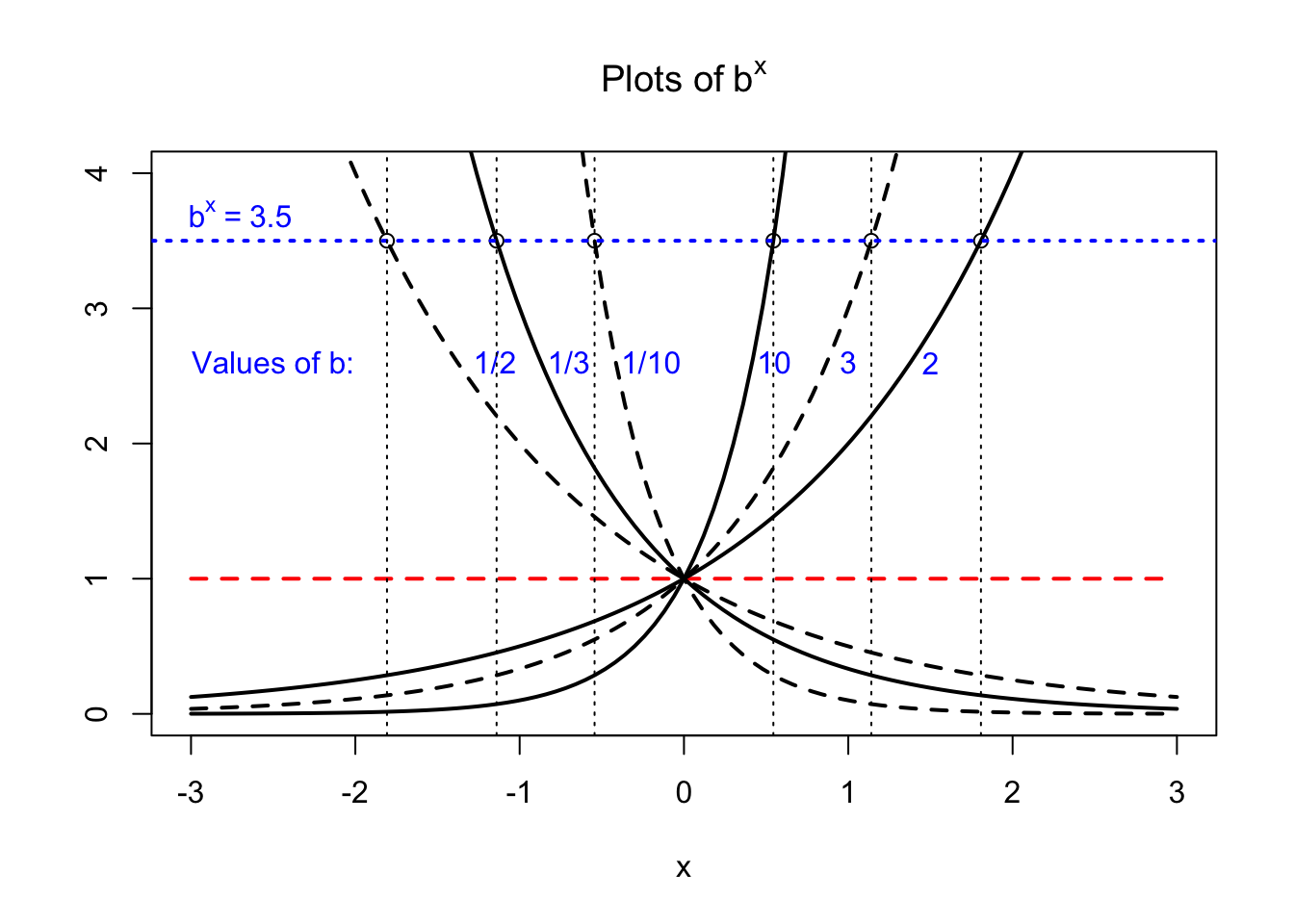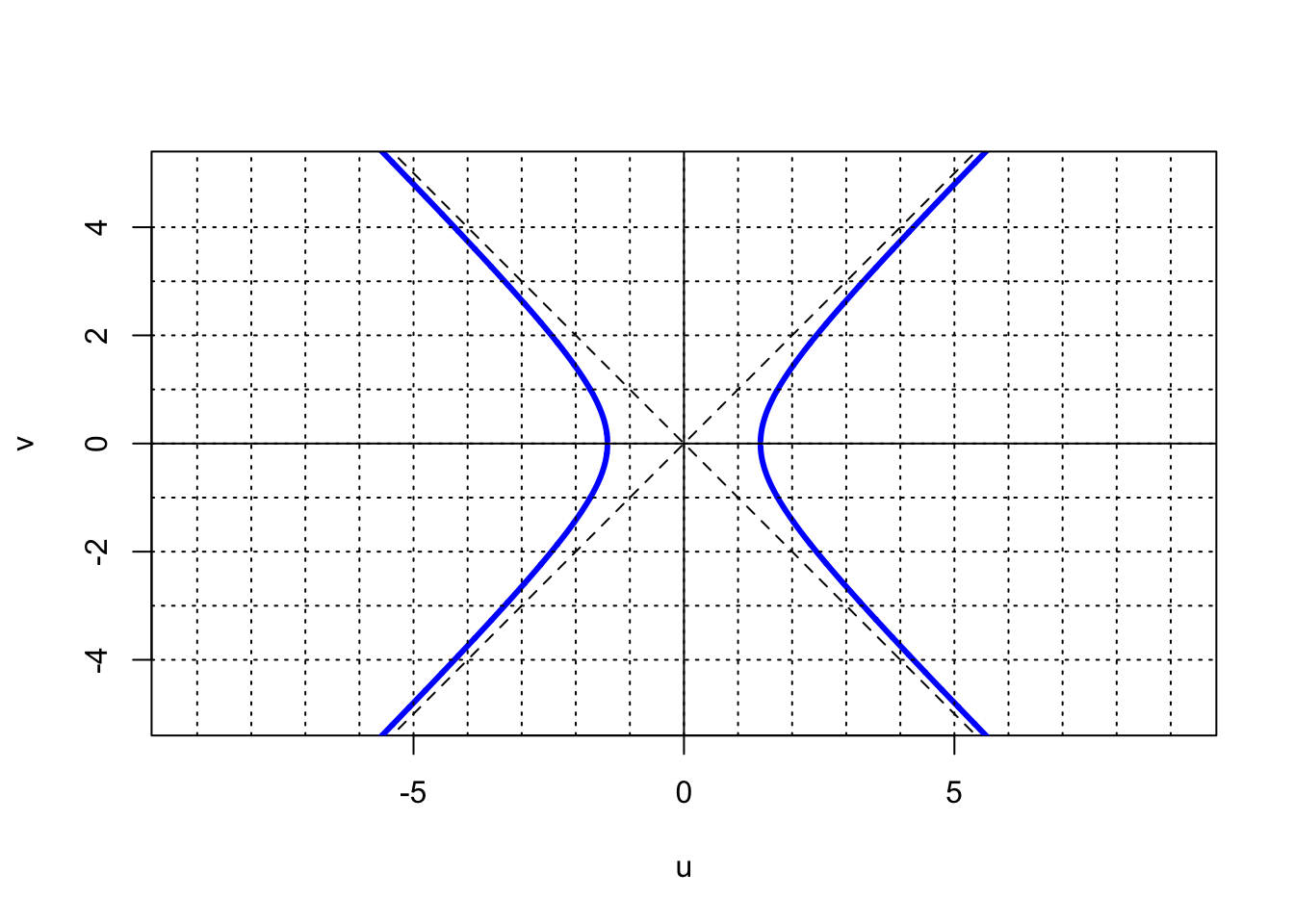## 2.2 The Natural Logarithm

We want to find a mathematical function $$f(x)$$ that can be evaluated in a straightforward way, and that has the properties of a logarithm that were discussed in the previous chapter. For instance, the function would need to have the following properties:

• The logarithm of 1 must zero for any choice of base. Hence, $$f(1) = 0.$$
• The function should be positive for values of $$x$$ greater than one, and negative for values less than one: $$f(x<1) < 0; ~~ f(x > 1) >0.$$
• As $$x$$ approaches zero from the positive side, the function should approach negatively infinite numbers: $$f(x)\rightarrow -\infty$$ as $$x\rightarrow 0.$$
• And, as $$x$$ gets more and more positive, the function should tend toward infinite numbers: $$f(x)\rightarrow +\infty$$ as $$x\rightarrow +\infty .$$
• Lastly, but perhaps most importantly, since the logarithm of a product of two numbers must be the sum of the logarithms of the two numbers, we must have: $$f(a\times b) = f(a) + f(b).$$

It turns out that the area under a specific geometrical figure, which can be accurately computed through the use of calculus, has the above properties and hence can be used to define a natural logarithm. This may seem like a very strange approach toward defining a logarithm which is, after all, an exponent applied to a base number, a seemingly unrelated mathematical exercise. But we’ll see that for one particular curve, these two operations have a remarkable connection and the power of calculus allows us to compute the answers in a straightforward way.

The curve in question is that of a hyperbola. In terms of the coordinates in a plane, $$u$$ and $$v$$, the equation of a hyperbola can be written as $$u\cdot v = 1$$ or, as a function of $$u$$, $$v(u) = 1/u$$.If we take the branch of the hyperbola for positive values of $$u$$ we find, after a bit of inspection, that our properties of a logarithm can be met by defining a new function that is evaluated by finding the $$area$$ between the hyperbolic curve and the horizontal axis. The area in question is shown below:The shaded area always starts at $$u=1$$ and stops at an arbitrary value $$x$$, and so yields a function of $$x$$, call it $$f(x)$$. This function, therefore, can be computed using calculus by performing the following integration: ${\cal Area} = f(x) = \int_1^x \frac{1}{u}\;du.$ This integral has the properties of a logarithm as listed above. First of all, it obeys the rule of a logarithm in that the logarithm of $$a\times b$$ is equal to the sum of the individual logarithms of $$a$$ and of $$b$$: $\begin{eqnarray*} f(a\times b) &=& \int_1^{ab} \frac{1}{u}\;du \\ &=& \int_1^{a} \frac{1}{u}\;du + \int_a^{ab} \frac{1}{u}\;du \\ &=& \int_1^{a} \frac{1}{u}\;du + \int_1^{b} \frac{1}{w}\;dw ~~~~~~~(w \equiv u/a)\\ &~& ~~ \\ &=& f(a) + f(b). \end{eqnarray*}$ Secondly, for $$x$$ = 1 the integration range will have zero length and hence $$f(1)$$ will be zero. For values of $$x<1$$ the integral will be negative, just as logarithms are negative for $$x<1$$. In addition, the integral goes to $$-\infty$$ as $$x$$ approaches 0 and to $$+\infty$$ as $$x$$ approaches $$+\infty$$. The function $$f(x)$$ given by the above integral obeys all the basic properties of a logarithm.

For this reason, this integral represents a natural logarithm and is given the designation $$\ln x$$ with the formal definition, $\ln x \equiv \int_1^x \frac{1}{u}\;du.$